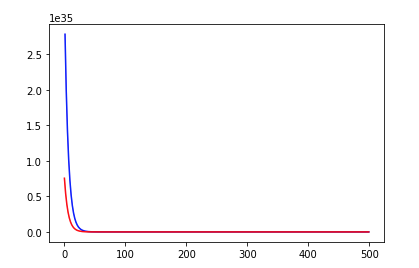# [Newbie] My training function returns empty losses. Am I doing it right?

I am trying to train a simple neural network for regression problem. Here X_train, y_train, X_valid, y_vlid are my X/y numpy arrays for training/validation. After running the training, my training and validation loss are empty (NaN). Here is my
model, criterion and training function, is there a problem with my function?

``````class Net_Reg(nn.Module):
def __init__(self):
super(Net_Reg, self).__init__()
self.fc1 = nn.Linear(15, 10)
self.fc2 = nn.Linear(10, 10)
self.fc3 = nn.Linear(10, 1)

def forward(self, x):
x = F.relu(self.fc1(x))
x = F.relu(self.fc2(x))
x = self.fc3(x)
return x

reg_model = Net_Reg()
criterion = torch.nn.MSELoss()
optimizer = optim.SGD(reg_model.parameters(), lr=0.01)

X_trn = torch.from_numpy(X_train).float()
y_trn = torch.from_numpy(y_train).float()
t_ds = TensorDataset(X_trn, y_trn)

X_val = torch.from_numpy(X_valid).float()
y_val = torch.from_numpy(y_valid).float()
v_ds = TensorDataset(X_trn, y_trn)

def reg_train(model, t_ds, v_ds, bs, epochs):

epoch_count = []
train_loss = []
val_loss = []

for epoch in range(epochs):

model.train()

train_running_loss = 0

t_outputs = model(t_inputs)
t_loss = criterion(t_outputs, t_labels)

train_running_loss += t_loss.item()

t_loss.backward()
optimizer.step()

epoch_count.append(epoch)
train_loss.append(train_running_loss)

model.eval()

val_running_loss = 0

v_outputs = model(v_inputs)
v_loss = criterion(v_outputs, v_labels)

val_running_loss += v_loss.item()

val_loss.append(val_running_loss)

print ("training loss: {:.4f}\nvalidation loss: {:.4f}".format(sum(train_loss)/len(t_ds),
sum(val_loss)/len(v_ds)))
plt.plot(epoch_count, train_loss, c='b')
plt.plot(epoch_count, val_loss, c='r')

return model``````

Could you print the `loss.item()` during training and have a look if it’s rising?
Also, could you print the shape of your target tensors?

thanks for the response. Here are the loss.item() and shape of target :

nan
torch.Size([4, 1])

Thanks for the information. It looks alright and I could successfully fit some random input:

``````X_trn = torch.randn(100, 15)
y_trn = torch.randn(100, 1)
t_ds = TensorDataset(X_trn, y_trn)

X_val = torch.randn(100, 15)
y_val = torch.randn(100, 1)
v_ds = TensorDataset(X_trn, y_trn)
``````

Could you try to lower your learning rate to `1e-3` and try it again?

learning rate helps is a sense that I don’t get NaN but now the loss is astonishingly large. After 500 epochs here are the loss:

training loss: 77571602712294141286416384.0000
validation loss: 30277167064551056736256.0000

How does the loss curve look like?
Is it decreasing at all or just rising?
In the latter case, could you try to lower the learning rate even more?

Also, what kind of data are you using?
What is the min, max, mean, std of your data?
Normalizing might help, if you are dealing with a large range of values.

The data are frequency settings on an instrument which correlates with the target.

X.std(), X.max(), X.min()
(1110.2270327891836, 8407.1, 3003.7)

The losses I reported previously was with learning rate of 0.001 and epochs=500. If i increase the epochs to 2000, the loss just go up to inf. If i decrease the learning rate to 0.0001 and keep the epoch at 500, the loss are still large . here is the graph:

``````X = X - X.mean()
X = X / X.std()
``````

Also, if your target has a similar range, you could also try to normalize it for training and denormalize it to get the “real” predictions.

Thanks for the suggestion; normalization does help but still the loss is huge; see below:

``````X_new.mean(), X_new.std(), X_new.max(), X_new.min()
(-1.443642e-16, 0.999999, 0.953241, -3.913690)
``````My y range is 0-4 and so i did not normalize it. I then tried classification (with 5 classes); the validation accuracy is now 0.58 (with normalization) … I was wondering if there is any other things i can try to minimize/improve the loss/accuracy?

I tried learning rate scheduler with cosine annealing and tried different learning rate but the improvement is insignificant.

Are you sure you are passing the normalized data to your model?
If the new mean values is approx. 0 and the target is in range `[0, 4]`, the loss shouldn’t be at >1e35.
Could you print a sample output of the first data batch you are feeding to the model?

sorry, I didn’t use the normalized X into dataset. With normalized ds, here is the training/validation loss after 2 epochs:

training loss: 0.2389
validation loss: 0.2239

here is the outputs of the first training data batch:

tensor([[2.2860],
[2.0087],
[2.1482],